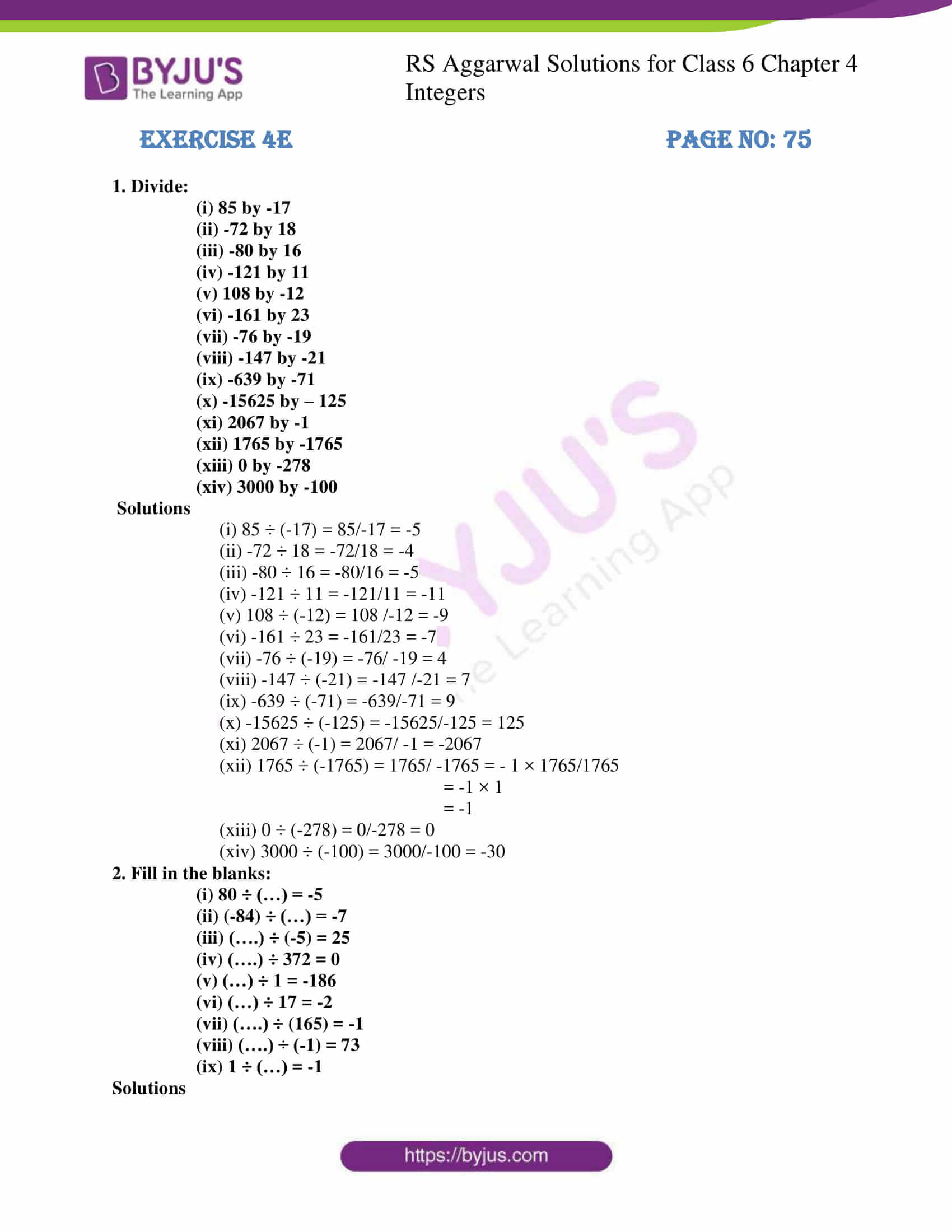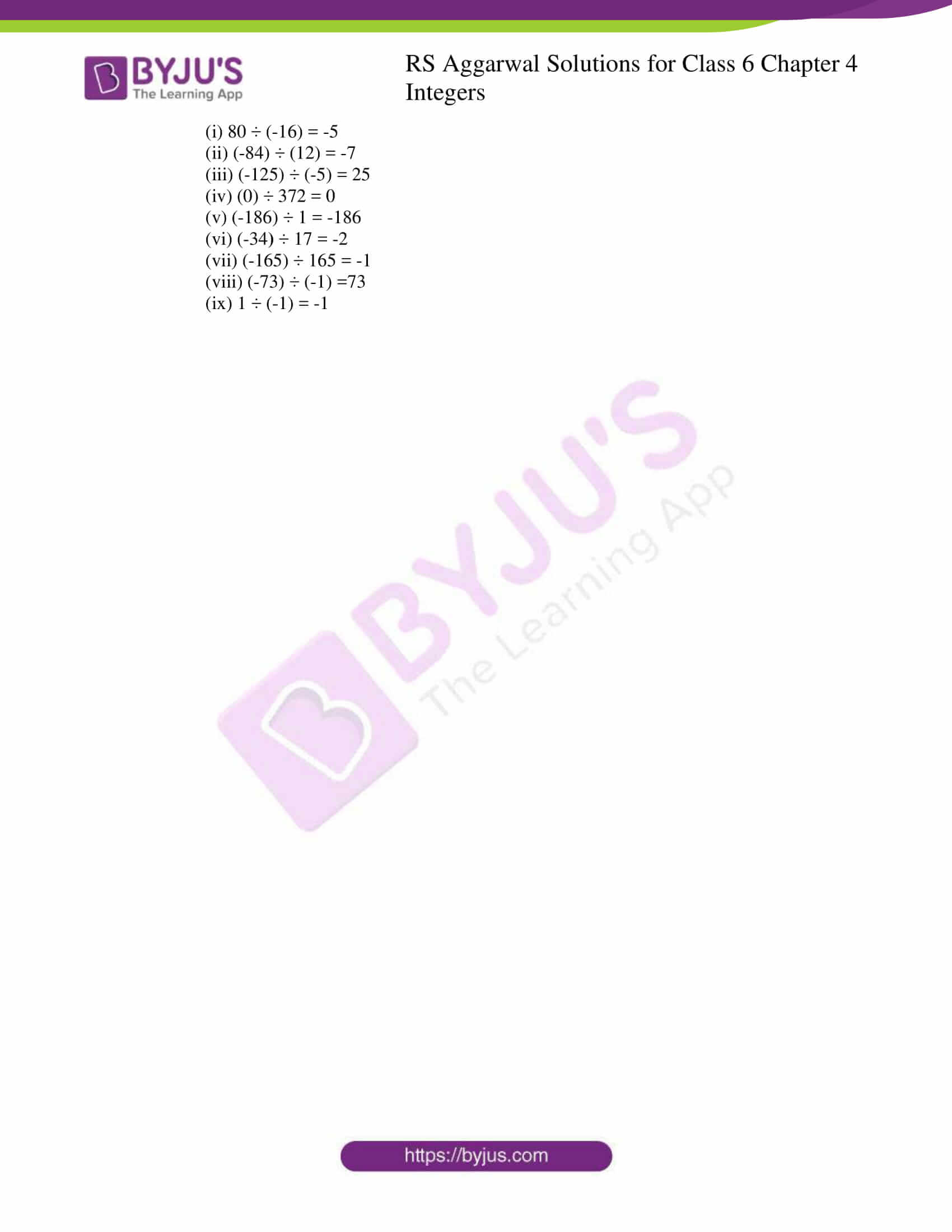# RS Aggarwal Solutions for Class 6 Chapter 4 Integers Exercise 4E

RS Aggarwal Solutions for Class 6 Chapter 4 Integers Exercise 4E are here in PDF for students to practice. The solutions created by faculty at BYJU’S can make students understand the subject in a better way. The solutions are very accurate and help students in completing their homework without any obstacles. Students can refer and learn more about this exercise by practicing RS Aggarwal Solutions, which are given in PDF here.

## Download PDF of RS Aggarwal Solutions for Class 6 Chapter 4 Integers Exercise 4E## Access answers to Maths RS Aggarwal Solutions for Class 6 Chapter 4 Integers Exercise 4E

1. Divide:

(i) 85 by -17

(ii) -72 by 18

(iii) -80 by 16

(iv) -121 by 11

(v) 108 by -12

(vi) -161 by 23

(vii) -76 by -19

(viii) -147 by -21

(ix) -639 by -71

(x) -15625 by – 125

(xi) 2067 by -1

(xii) 1765 by -1765

(xiii) 0 by -278

(xiv) 3000 by -100

Solutions

(i) 85 ÷ (-17) = 85/-17 = -5

(ii) -72 ÷ 18 = -72/18 = -4

(iii) -80 ÷ 16 = -80/16 = -5

(iv) -121 ÷ 11 = -121/11 = -11

(v) 108 ÷ (-12) = 108 /-12 = -9

(vi) -161 ÷ 23 = -161/23 = -7

(vii) -76 ÷ (-19) = -76/ -19 = 4

(viii) -147 ÷ (-21) = -147 /-21 = 7

(ix) -639 ÷ (-71) = -639/-71 = 9

(x) -15625 ÷ (-125) = -15625/-125 = 125

(xi) 2067 ÷ (-1) = 2067/ -1 = -2067

(xii) 1765 ÷ (-1765) = 1765/ -1765 = – 1 × 1765/1765

= -1 × 1

= -1

(xiii) 0 ÷ (-278) = 0/-278 = 0

(xiv) 3000 ÷ (-100) = 3000/-100 = -30

2. Fill in the blanks:

(i) 80 ÷ (…) = -5

(ii) (-84) ÷ (…) = -7

(iii) (….) ÷ (-5) = 25

(iv) (….) ÷ 372 = 0

(v) (…) ÷ 1 = -186

(vi) (…) ÷ 17 = -2

(vii) (….) ÷ (165) = -1

(viii) (….) ÷ (-1) = 73

(ix) 1 ÷ (…) = -1

Solutions

(i) 80 ÷ (-16) = -5

(ii) (-84) ÷ (12) = -7

(iii) (-125) ÷ (-5) = 25

(iv) (0) ÷ 372 = 0

(v) (-186) ÷ 1 = -186

(vi) (-34) ÷ 17 = -2

(vii) (-165) ÷ 165 = -1

(viii) (-73) ÷ (-1) =73

(ix) 1 ÷ (-1) = -1